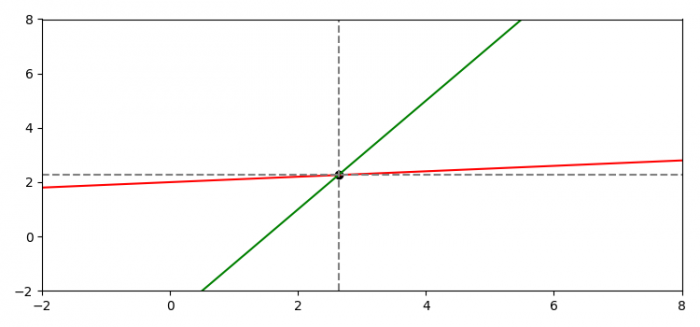# Plot horizontal and vertical lines passing through a point that is an intersection point of two lines in Matplotlib

To plot horizontal and vertical lines passing through a point, we can take the following steps

• Set the figure size and adjust the padding between and around the subplots.
• Create two lines using slopes (m1, m2) and intercepts (c1 and c2). Initialize the slopes and intercepts values.
• Create x data points using numpy.
• Plot x, m1, m2, c2 and c1 data points using plot() method.
• Using intercepts and slopes values, find the point of intersection.
• Plot horizontal and vertical lines with dotted linestyle.
• Plot xi and yi points on the plot
• To display the figure, use show() method.

## Example

import matplotlib.pyplot as plt
import numpy as np
plt.rcParams["figure.figsize"] = [7.50, 3.50]
plt.rcParams["figure.autolayout"] = True
m1, c1 = 0.1, 2.0
m2, c2 = 2.0, -3.0
x = np.linspace(-10, 10, 500)
plt.plot(x, x * m1 + c1, 'red')
plt.plot(x, x * m2 + c2, 'green')
plt.xlim(-2, 8)
plt.ylim(-2, 8)
xi = (c1 - c2) / (m2 - m1)
yi = m1 * xi + c1
plt.axvline(x=xi, color='gray', linestyle='--')
plt.axhline(y=yi, color='gray', linestyle='--')
plt.scatter(xi, yi, color='black')
plt.show()

## Output